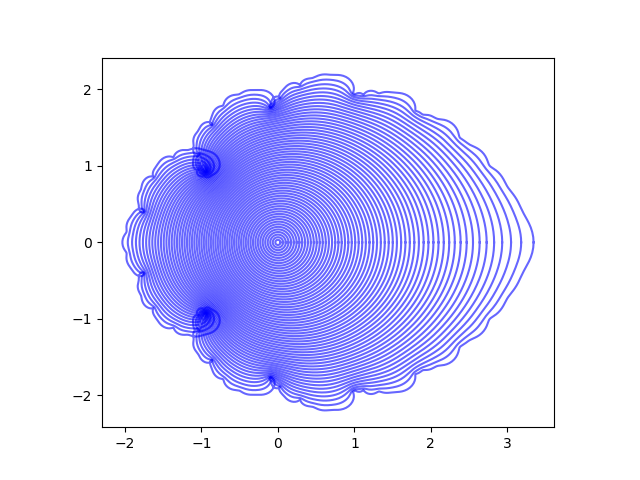# Fibonacci numbers and ingrown bark

The previous post looked at the images of concentric circles under functions defined by power series. The terms of these series have the form

zθ(n) / θ(n)

where θ(n) is a rapidly increasing function of n. These series are thin (technically, lacunary) because all the terms between values of θ(n) are missing.

The previous post used factorials and powers of 2 as the thinning function. This post uses Fibonacci numbers. It differs from the previous post by using more circles and by making all the circles the same color rather than using matplotlib’s default colors.

Without further ado, here’s the plot.It looks sorta like a tree with ingrown bark.

The code to produce the plot was the same as the code in the previous post with the following changes.

```    def fib(n):
phi = (1 + 5**0.5)/2
return int(phi**n/5**0.5 + 0.5)

for r in linspace(0, 1, 60):
z = f(fib, r*exp(1j*theta))
plt.plot(z.real, z.imag, 'b', alpha=0.6)
```

The function `fib` above uses Binet’s formula to compute Fibonacci numbers.